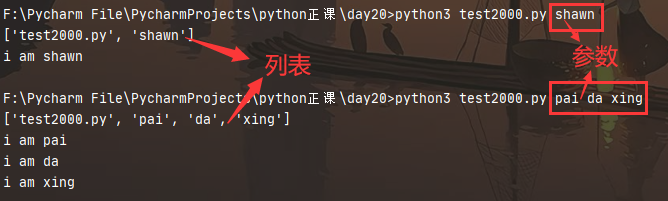# 43 sys 模块

77次阅读

## 引入

### 1. 什么是 sys 模块

• sys 模块是与 Python 解释器交互的一个 接口
• 该模块提供对解释器使用或维护的一些 变量 的访问和 获取
• 它提供了许多函数和变量来处理 Python 运行时环境的不同部分

## 一. 常见用法介绍

### 1.sys.argv

• 实现从程序的外部向程序传递参数
• 返回的是一个列表, 第一个元素是程序文件名, 后面的元素都是程序外部传入的参数
• 用法演示 :
``````import sys

print(sys.argv)
test = sys.argv
if test == "shawn":
print(f"i am {test}")
else:
for i in range(1,len(sys.argv)):  # 多个参数的时候将其遍历打印
print(f"i am {sys.argv[i]}")
``````
• 在终端运行时传入一个参数或多个参数### 2.sys.path

• 获取指定模块搜索路径的字符串集合, 返回的是一个 列表 (表示可以使用 append 或者 insert)
• 可以将写好的模块放入某个路径下, 然后将这个路径添加到 sys.path 里面, import时就可以找到
• 用法演示 :
``````import sys

print(sys.path)
'''['F:\\Pycharm File\\PycharmProjects\\python 正课 \\day17',\'F:\\Pycharm File',\'J:\\PyCharm 2020.2.3\\plugins\\python\\helpers\\pycharm_display',\'K:\\python37\\python37.zip',\'K:\\python37\\DLLs',\'K:\\python37\\lib',\'K:\\python37',\'K:\\python37\\lib\\site-packages',\'J:\\PyCharm 2020.2.3\\plugins\\python\\helpers\\pycharm_matplotlib_backend']'''
🔰可以发现是一个列表 (代表着可以使用 "append" 功能向里面添加路径)
🔰第一个路径是当前执行文件所在的目录
🔰第二个是整个项目的路径, "PyCharm" 的优化 (在别的编辑器上没有, 可以忽略)
🔰第四个是 "zip" 格式压缩包, 其实它是 python 解释器, 里面放的是内置以及标准库模块
🔰其他的一些都是第三方库或者自定义下载的模块
``````
• 添加路径
``````import sys
sys.path.append("[文件路径]")

🔰例:
import sys
sys.path.append("F:\Pycharm File\PycharmProjects\python 正课 \day10")
print(sys.path[-1])  # F:\Pycharm File\PycharmProjects\python 正课 \day10
``````

### 3.sys.exit(n)

• 退出程序, 正常退出时 sys.exit(0), 其他为异常退出
• 一般情况下运行到主程序尾部, 解释器就正常退出了, 但如果想要中途退出, 就可以调用 sys.exit 函数

ps : exit()运用在交互式 shell 中，而 sys.exit 主要用于程序中，二者功能类似

### 4.sys.version

• 获取 Python 解释程序的版本信息
``````import sys

print(sys.version)
# 3.7.4 (tags/v3.7.4:e09359112e, Jul  8 2019, 20:34:20) [MSC v.1916 64 bit (AMD64)]
``````

### 5.sys.platform

• 返回操作系统平台名称
``````import sys

print(sys.platform)  # win32
``````

### 6.sys.maxint 与 sys.maxsize

• 最大的 int 值
• Python3 中删除了 sys.maxint 常量, 因为不再对整数值进行限制
``````import sys

print(sys.maxint)
# AttributeError: module 'sys' has no attribute 'maxint'
print(sys.maxsize)
# 9223372036854775807
``````

### 7.sys.getdefaultencoding()

• 获取解释器默认编码
``````import sys

print(sys.getdefaultencoding())  # utf-8
``````

### 8.sys.getfilesystemencoding()

• 获取内存数据存到文件里的默认编码
``````import sys

print(sys.getfilesystemencoding())  # utf-8
``````

### 9.sys.getrecursionlimit()

• 获取最大递归层数
``````import sys

print(sys.encursionlimit())  # 1000
``````

### 10.sys.setrecursionlimit()

• 设置最大递归层数
``````import sys

sys.setrecursionlimit(1314)
print(sys.getrecursionlimit())  # 1314
``````

• 标准输入
``````import sys

print(res)
songha  # 这是控制台输入的字符串
songha  # 这是 print 输出的字符串 "songha\n"
``````

### 12.sys.stdout.write("xxx")

• 标准输出 (相比较 print 能更好的控制输出)
``````import sys

sys.stdout.write('shawn\n')  # Shawn
``````
• 模拟简单进度条动态加载
``````import sys , time

for i in range(50):
sys.stdout.write("█")
time.sleep(0.1)
sys.stdout.flush()  # 刷新显示到屏幕上
# ██████████████████████████████████████████████████ 动态的加载进度条
``````

## 二. 模拟进度条打印

``````🔰知识储备
'%-50s'  # "%": 字符串格式化; "-": 左对齐, 数字和字符串默认都是右对齐; "50": 代表我设置的宽度
'r'  # 表示让光标跳到行首

🔰"print" 模拟输出进度条效果, 宽度是 "40", 传入的是 "█"
print("▌%-40s▌" %'█')
print("▌%-40s▌" %'██')
print("▌%-40s▌" %'███')
print("▌%-40s▌" %'████')
print("▌%-40s▌" %'█████')
''' 输出
▌█                                       ▌
▌██                                      ▌
▌███                                     ▌
▌████                                    ▌
▌█████                                   ▌
'''

🔰打印百分比 "%", 第一个是传值, 第三个是取消第二个百分号的特殊意义
print('%s%%' %(10))
print('%s%%' %(30))
print('%s%%' %(80))
print('%s%%' %(100))
''' 输出
10%
30%
80%
100%
'''

🔰使用参数来实现可变宽度
print("▌%%-%ds▌" % 40)  #  "▌%-40s▌"
print(("▌%%-%ds▌" % 40) % "██")
print(("▌%%-%ds▌" % 40) % "███")
print(("▌%%-%ds▌" % 40) % "████")
print(("▌%%-%ds▌" % 40) % "█████")

🔰开始实现:
def progress(percent, symbol='█', width=40):
if percent > 1:     # 超过 100% 的时候让其停在 1
percent = 1     # 可以避免进度条溢出
show_progress = ("▌%%-%ds▌" % width) % (int(percent * width) * symbol)
print("\r%s %.2f%%" % (show_progress, percent * 100), end='')

def plan():
data_size = 1025                  # 传输数据
recv_size = 0                     # 初始值为 0
while recv_size < data_size:
time.sleep(0.1)               # 模拟数据的传输延迟
recv_size+=150                # 每次收 150

percent=recv_size / data_size # 接收的比例
progress(percent, width=40)   # 进度条的宽度 40

plan()
# ▌████████████████████████████████████████▌ 100.00%
``````